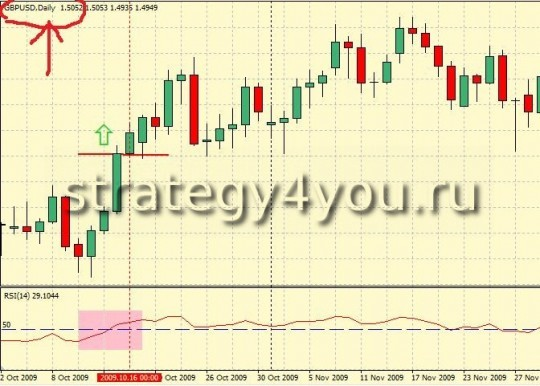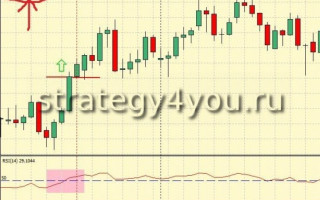# Forex Strategy H4 Fibonacci Method

Forex Strategy H4 Fibonacci Method — multi-currency, trend forex strategy, trading in which is the time interval H4, Auxiliary lights — 2 moving average indicator RSI, the main tool — Fibonacci (Fibonacci).

As some professional traders: «Fibonacci — the only» indicator «, which predicts the point of a trend reversal in the financial markets!

That is why the tool is so popular Fibonacci forex trading, and in all financial markets — it is indeed one of the best tools that can predict a trend reversal point (or a temporary rebound), so it helps Most of traders to profit by his skillful use of the forex market.

Let’s look at forex strategy, in which the Fibonacci play quite a role. So to trade forex strategy H4 Fibonacci Method, must be installed on schedule for the chosen currency pair (which, again, can be any).

The following forex indicators:

• Daily chart (D1) — Oscillator Relative Strength Index with a period of 14 to apply to close (RSI (14)), as well as add a level of 50
• schedule H4 — Exponential Moving Average EMA (100), applied to a close — the color green
• schedule H4 — simple moving average SMA (150), applied to a close — the color red
• And also we need a tool Fibonacci levels 0.382, 0.618, 0.236 and 0.764.## Conclude a deal to buy if:

1. RSI (14) on the daily chart was closed and is located above the level 50 — trade only in this direction! Transactions on sale does not conclude!

2. Green Moving Average EMA (100) crossed the red moving average, SMA (150) from the bottom up — will sell only in this direction!

3. find the two closest extremum — minimum and maximum distance between them is at least 100 points. And no matter where the trend between the extremes — up or down.

4. Fibonacci stretch according to the extrema.

5. We wait until the price comes to a corridor between the Fibonacci levels 38.2% and 61.8%

6. And then waiting for the price out of these levels and closes the first candle (candle complete — including the tail) above the level of 76,4% (if we stretched the Fibonacci down — corrective movement to the rising trend), or 23,6% (if we Fibonacci stretched from the bottom up — the direction of travel of the upward trend).
7. Stop-lossset at least at the level of 100 points, or under the nearest local minimum.

8. Profit target set at a distance of 161.8% of the extendedFibonacci sequence by which you have entered the market. Ifdesired, you can use the trailing stop or close the deal at a distance of 261.8%, etc.

For transactions on SELL check conditions.